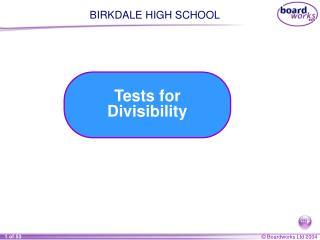# Tests for Divisibility - PowerPoint PPT PresentationDownload PresentationTests for Divisibility

Tests for DivisibilityDownload Presentation## Tests for Divisibility

- - - - - - - - - - - - - - - - - - - - - - - - - - - E N D - - - - - - - - - - - - - - - - - - - - - - - - - - -
##### Presentation Transcript

1. BIRKDALE HIGH SCHOOL Tests for Divisibility

2. Divisibility by 2 Is 367 908 divisible by 2? Is 367 908 367 908 is divisible by 2 if 2 divides into it exactly without leaving any remainders. We can tell if a number is divisible by 2 by looking at the last digit in the number. A number is divisible by 2 if the last digit is 0, 2, 4, 6 or 8. Yes, 367 908 is divisible by 2. It is an even number.

3. Divisibility by 3 Is 35 841 divisible by 3? We can tell if a number is divisible by 3 by adding together its digits. This is called finding the digit sum. A number is divisible by 3 if the digit sum is divisible by 3. The digit sum of 35 841 is 3 + 5 + 8 + 4 + 1 = 21 21 is divisible by 3. Yes, 35 841 is divisible by 3.

4. Divisibility by 4 Is 1 934 274 divisible by 4? Is 1 934 274 We can tell if a number is divisible by 4 by looking at the last two digits in the number. A number is divisible by 4 if the last two digits are divisible by 4. A two-digit number is divisible by 4: • if it ends in 0, 4 or 8 and the first digit is even • if it ends in 2 or 6 and the first digit is odd. No, 1 934 274 is not divisible by 4.

5. Divisibility by 5 Is 231 030 divisible by 5? Is 231 030 We can tell if a number is divisible by 5 by looking at the last digit in the number. A number is divisible by 5 if the last digit is 0 or 5. Yes, 231 030 is divisible by 5.

6. Divisibility by 6 Is 53 702 divisible by 6? We can tell if a number is divisible by 6 by looking at its last digit and finding its digit sum. A number is divisible by 6 if it is divisible by both 2 and 3. The last digit in 53 702 is a 2 and so it is divisible by 2. The digit sum of 53 702 is 5 + 3 + 7 + 0 + 2 = 17 17 is not divisible by 3 and so 53 702 is not divisible by 3. 53 703 is divisible by 6 only if it is divisible by both 2 and 3. No, 53 702 is not divisible by 6.

7. Divisibility by 8 Is 63 072 divisible by 8? We can tell if a number is divisible by 8 by halving it and checking for divisibility by 4. A number is divisible by 8 if half of it is divisible by 4. Half of 63 072 is 31 536 31 536 Look at the last two digits. 36 is divisible by 4. Yes, 63 072 is divisible by 8.

8. Divisibility by 9 Is 6 873 975 divisible by 9? We can tell if a number is divisible by 9 by finding its digit sum. A number is divisible by 9 if its digit sum is divisible by 9. The digit sum of 6 873 975 is 6 + 8 + 7 + 3 + 9 + 7 + 5 = 45 45 is divisible by 9. Yes, 6 873 975 is divisible by 9.

9. Divisibility by 10 Is 6 311 289 divisible by 10? Is 6 311 289 We can tell if a number is divisible by 10 by looking at the last digit in the number. A number is divisible by 10 if the last digit is 0. No, 6 311 289 is not divisible by 10.

10. Divisibility by larger numbers Is 214 875 divisible by 15? We test for divisibility by some larger numbers by using two simpler tests. A number is divisible by 15 if it is divisible by both 3 and 5. The digit sum of 214 875 is 2 + 1 + 4 + 8 + 7 + 5 = 27 27 is divisible by 3 and so 214 875 is divisible by 3. The last digit in 214 875 is a 5 and so it is also divisible by 5. Yes, 214 875 is divisible by 15.

11. Divisibility by larger numbers We can check for divisibility by other larger numbers by testing for divisibility by factors of the number. For example, A number is divisible by 12 if it is divisible by both 3 and 4. A number is divisible by 15 if it is divisible by both 3 and 5. A number is divisible by 18 if it is divisible by both 2 and 9. A number is divisible by 20 if it is divisible by both 4 and 5. A number is divisible by 24 if it is divisible by both 3 and 8. A number is divisible by 30 if it is divisible by both 3 and 10.

12. Using divisibility to find multiples